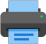Lista de exercícios do ensino médio para impressãoSelect all.
print selected exercises
Three points are given in the (x,y)-plane: $\;A=(1,2)\;$, $\;B=(2,-2)\;$ and $\;C=(4,3)\;$. The equation of the line passing through the point $\;A\;$ and the midpoint of the segment $\;\overline{BC}\;$ is:
a)
$3x + 4y = 11$
b)
$4x + \dfrac{7}{2}y = 11$
c)
$x + 3y = 7$
d)
$3x + 2y = 7$
e)
$x + 2y = 5$